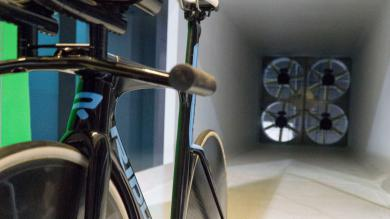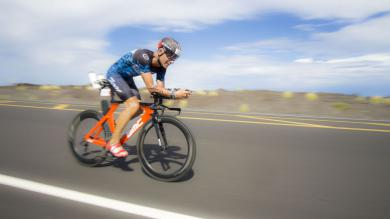# Power to Speed Calculator

Choose temperature, altitude, tires, CdA, drivetrain efficiency and calculate the speed for a given power output.

## Speed

0 km/h

The speed of a cyclist depends on a number of factors. In order to move at a certain speed, a cyclist has to overcome a number of forces. In most riding scenarios the aerodynamic drag accounts for the largest part of these forces. It is followed by the gravitational forces, the rolling resistance of the tires and mechanical losses of the drivetrain.

## The aerodynamic drag

In recent years the importance of aerodynamics was recognized by most professional and many amateur cyclists. Back in 1989 Greg Lemond beat Laurent Fignon in the final stage time trial of the Tour de France by 58 seconds using an aerodynamic helmet and aerobars. These 58 seconds were enough to win the Tour with a time advantage of only 8 seconds. At the same time this dramatic final stage proved the advantage of an aerodynamic position and equipment to a large audience.

The main factors of aerodynamic drag are the air density (which depends on temperature and altitude) and of course the size and shape of the cyclist and his bike. To define the latter the drag area CdA is commonly used. CdA stands for the product of the frontal area of rider and bike, named A, and the drag coefficient cd (often also cw). Since aerodynamic optimizations, for example changes in a rider’s position) often affect the riders shape as well as his frontal area, the combination of the latter, the CdA, is a popular key figure to measure, compare and optimize the aerodynamic qualities of cyclists.

Ignoring the other resistances for the moment, the power needed to overcome the aerodynamic drag is calculated by:

Powerdrag = 0.5 * CdA * Air density * Speed3

## The rolling resistance

Who would have thought it, but the rolling resistance mainly depends on the tires (pressure, compound and profile) and the systems weight (rider+bike). The CRR coefficient is commonly used to characterise a tires rolling resistance. Here I strongly recommend you to take a look at the website www.bicyclerollingresistance.com, were you can find a large number of tire-tests together with CRR coefficients for commonly used tire pressures.

Again, ignoring the other resistances for the moment, the power needed to overcome the rolling resistance is calculated by:

Powerrolling = 9.8067 * Weight * CRR * Speed

Here the number 9.8067 represents the gravitational acceleration with the unit m/s2 which I omitted in the formula above for reasons of simplicity. Furthermore note, that this formula is only correct on a level track, since only in this case the gravitational forces are directed entirely towards the road.

## Mechanical losses

Road bike drivetrains (with derailleur gears) can have efficiencies up to 98%. The chain lubrication, bearings and the gear in use can affect the efficiency of a drivetrain significantly and in the worst case can lead to drivetrain efficiencies as low as 93%.

Most powermeters measure the power at the front chainrings or the pedals. In case you use a powermeter like this and you want to compare the power that you enter in the above calculator, to the Watts you see on your bicycle computer, you should enter a number below 98% (typically 96-97.5% for amateur riders) for the assumed mechanical losses of your drivetrain. If you use a hub powermeter you can enter 100% since the measured power is not affected by losses in the drivetrain.

## Gravitational forces

As mentioned in the section about the rolling resistance the calculator above assumes a perfectly level track. Therefore, the gravitational forces only appear in the rolling resistance part of the overall speed calculation. For the sake of completeness, I would like to mention the gravitational forces, which act when riding up or down a hill.

First of all, we have to take a look at the term gradient. The gradient of a road is usually stated in percent and indicates the rise over run ratio. On a road with a gradient of 10% you gain 10m in altitude while covering a horizontal distance of 100m. In order to determine the gravitational forces, we are interested in the angle of inclination which is calculated by:

angle of inclination = arctan(gradient in percent / 100)

Let us call the angle of inclination α in the following.

Now let us get back to the gravitational force. While ascending or descending the gravitational forces do not act perpendicular to the road anymore. One part of the force still pushes the rider towards the road, while the other part either pushes him forwards (in descend) or backwards (in ascend).

Therefore, the rolling resistance changes to

Powerrolling = 9.8067 * Weight * cos(α) * CRR * Speed,

while we can calculate the power related to the forces “pushing” or “pulling” us on a hill with

Powergradient = 9.8067 * Weight * sin(α) * Speed.

Note that angle of inclination α usually has a value between -20° and +20 (for descending or respectively ascending the steepest road in the world with a gradient of around 35%). Sin(α) can therefore be either positive or negative and likewise Power_gradient for riding up- or respectively down a hill.

## The wind

Now we talked a lot about aerodynamics, gravity and overcoming the forces of nature and did not mention the wind once. So far, we assumed perfect cycling conditions without a single breeze.

If we take the wind into account, what a surprise, we have to make some adjustments to the Powerdrag formula mentioned above. But in order to understand these changes we have to go a step back and look a little bit closer on how we established the power formulas in the first place.

In the introduction to this 'calculator-insights' I wrote that the rider has to overcome certain forces. Since force and power are not the same, I would like to outline their relation at this point.

Simply said power is defined as work over time, while work is defined as force times distance. This leaves us with:

Power = Force*Distance/Time (and Distance/Time = Speed)

Now let us go back to the aerodynamic drag which is defined as

Forcedrag = 0.5 * CdA * Air density * Speed^2

By multiplying Force_drag with the speed we arrive at the formulation

Powerdrag = 0.5 * CdA * Air density * Speed^3

Now let us assume there is some headwind with a certain speed. This results in

Forcedrag wind = 0.5 * CdA * Air density * (Speed + Headwind Speed)^2.

The corresponding power formula reads

Powerdrag wind = 0.5 * CdA * Air density * (Speed+ Headwind Speed)^2 * Speed.

(Ignoring all other forces) one might assume, that riding at a speed of 30km/h in windless conditions requires the same power as riding at 20km/h against a headwind with the speed of 10 km/h.

The first leads to

Powerno wind = 0.5 * CdA * Air density * (30/3,6 )^3 = 289,35 m^3/s^3 * CdA * air density

while the other leads to

Powersome wind = 0.5 * CdA * Air density * (30/3,6 )^2 * 20/3,6 = 192,90 m^3/s^3 * CdA * air density

So the intuitive approach leaves us to a wrong result in this case.

## Related### How to qualify for the Ironman Hawaii 2020### CdA Calculator### Simple Power to Speed Calculator### Best countries at the Ironman Hawaii 2018### Hawaii qualification and slot distribution